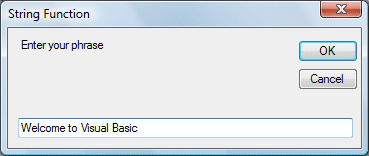# Lesson 13: String Manipulating Functions

We have learned about the basic concept of function as well as the MsgBox and InputBox functions in Lesson 12. I. In fact, I have already shown you a few string manipulation functions in Lesson 8, they are the Len function, the Left function and the Right Function. In this lesson, we will learn other string manipulation functions.

### 13.1 The Mid Function

The Mid function is used to retrieve a part of text form a given phrase. The syntax of the Mid Function is

```		Mid(phrase, position,n)
```

where

• phrase is the string from which a part of text is to be retrieved.
• position is the starting position of the phrase from which the retrieving process begins.
• n is the number of characters to retrieve.

#### Example 13.1

Private Sub Button1_Click(ByVal sender As System.Object, ByVal e As System.EventArgs) Handles Button1.Click
Dim myPhrase As String
Label1.Text = Mid(myPhrase, 2, 6)
End Sub

* In this example, when a user clicks the command button, an input box will pop up asking the user to input a phrase. After a phrase is entered and the OK button is pressed, the label will show the extracted text starting from position 2 of the phrase and the number of characters extracted is 6. The diagrams are shown below:### 13.2 The Right Function

The Right function  extracts the right portion of a phrase. The syntax is

Microsoft.Visualbasic.Right ("Phrase", n)

Where n is the starting position from the right of the phase where the portion of the phrase is going to be extracted. For example:

Microsoft.Visualbasic.Right ("Visual Basic", 4) = asic

Example 13.2: The following code extracts the right portion any phrase entered by the user.

Private Sub Button1_Click (ByVal sender As System.Object, ByVal e As System.EventArgs) Handles Button1.Click

Dim myword As String

myword = TextBox1.Text

Label1.Text = Microsoft.VisualBasic.Right (myword, 4)

End Sub

### 13.3 The Left Function

The Left function extracts the left portion of a phrase. The syntax is

`Microsoft.Visualbasic.Right ("Phrase", n)`

Where n is the starting position from the left of the phase where the portion of the phrase is going to be extracted. For example:

`Microsoft.Visualbasic.Left("Visual Basic", 4) =asic`

#### Example 13.3: The following code extracts the left portion any phrase entered by the user.

```
Private Sub Button1_Click (ByVal sender As System.Object, ByVal e As System.EventArgs) Handles Button1.Click
Dim myword As String
myword = TextBox1.Text
Label1.Text = Microsoft.VisualBasic.Left
(myword, 4)
End Sub```

### 13.4 The Trim Function

The Trim function trims the empty spaces on both side of the phrase. The syntax is

`Trim("Phrase")`

For example, Trim ("    Visual Basic      ") = Visual basic

### Example 13.4

```Private Sub Button1_Click(ByVal sender As System.Object, ByVal e As System.EventArgs) Handles Button1.Click
Dim myPhrase As String
Label1.Text = Trim(myPhrase)
End Sub
```

### 13.5 The Ltrim Function

The Ltrim function trims the empty spaces of the left portion of the phrase. The syntax is

`Ltrim("Phrase")`

.For example,

Ltrim ("°     Visual Basic")= Visual basic

13.6 The Rtrim Function

The Rtrim function trims the empty spaces of the right portion of the phrase. The syntax is

Rtrim("Phrase")

.For example,

Rtrim ("Visual Basic      ") = Visual Basic

13.7 The InStr function

The InStr function looks for a phrase that is embedded within the original phrase and returns the starting position of the embedded phrase. The syntax is

Instr (n, original phase, embedded phrase)

Where n is the position where the Instr function will begin to look for the embedded phrase. For example

Instr(1, "Visual Basic"," Basic")=8

*The function returns a numeric value.

You can write a program code as shown below:

Private Sub Button1_Click(ByVal sender As System.Object, ByVal e As System.EventArgs) Handles Button1.Click

Label1.Text = InStr(1, "Visual Basic", "Basic")
End Sub

13.8 The Ucase and the Lcase Functions

The Ucase function converts all the characters of a string to capital letters. On the other hand, the Lcase function converts all the characters of a string to small letters.

The syntax is

Microsoft.VisualBasic.UCase(Phrase)

Microsoft.VisualBasic.LCase(Phrase)

For example,

Microsoft.VisualBasic.Ucase("Visual Basic") =VISUAL BASIC

font face="Arial" > Microsoft.VisualBasic.Lcase("Visual Basic") =visual basic

#### 13.9 The Chr and the Asc functions

The Chr function returns the string that corresponds to an ASCII code while the Asc function converts an ASCII character or symbol to the corresponding ASCII code. ASCII stands for ¡°American Standard Code for Insyntaxion Interchange¡±. Altogether there are 255 ASCII codes and as many ASCII characters. Some of the characters may not be displayed as they may represent some actions such as the pressing of a key or produce a beep sound. The syntax of the Chr function is

Chr(charcode)

and the syntax of the Asc function is

Asc(Character)

The following are some examples:

Chr(65)=A, Chr(122)=z, Chr(37)=% ,

Asc("B")=66, Asc("&")=38

sage box. The Style Value  will determine what type of command buttons appear on the message box, please refer Table 10.1 for types of command button displayed. The Title argument will display the title of the message board.